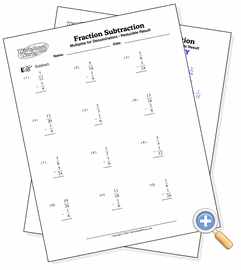# With Uncommon Denominators

## Fraction SubtractionDevelop subtraction skills with simple fractions having uncommon denominators

These problems involve the subtraction of simple fractions which do not have the same denominator. This requires the student to change one or both of the fractions so that the denominators are the same before subtracting. The first denominators option in the form below makes it so that one denominator is always a multiple of the other, meaning that the student only needs to change one fraction.